/ ggplot2 Public

# Feature request: Scaled densities/counts in 2d density/bins plots.#2679

Closed
opened this issue Jun 1, 2018 · 1 comment
Closed

# Feature request: Scaled densities/counts in 2d density/bins plots. #2679

opened this issue Jun 1, 2018 · 1 comment
Labels
feature a feature request or enhancement

### bjreisman commented Jun 1, 2018

 2d density plots are one of the most common data-visualizations used to display flow cytometry data, and the `geom_bin2d` and `geom_hex` and `geom_density_2d` geoms are excellent for making these plots. However, when facetting 2d density plots, there isn't a straightforward way to set the scale such that the highest point of each plot is the same - the convention in my field. geom_density (the 1d version) has an option to set `fill = ..scaled..` which rescales all groups to a maximum of 1. Would you consider adding such an option to `geom_bin2d` and `geom_hex` (and `stat_bin2d`/`stat_binhex`)? ``````library(ggplot2) library(dplyr) library(viridis) #2d density plot colored by level plot.density2d_level <- diamonds %>% ggplot(aes(x=x, y= depth)) + stat_density_2d(aes(fill = stat(level)), geom = "polygon", n = 100 , bins = 10) + facet_wrap(clarity~.) + scale_fill_viridis(option = "A") plot.density2d_level ``````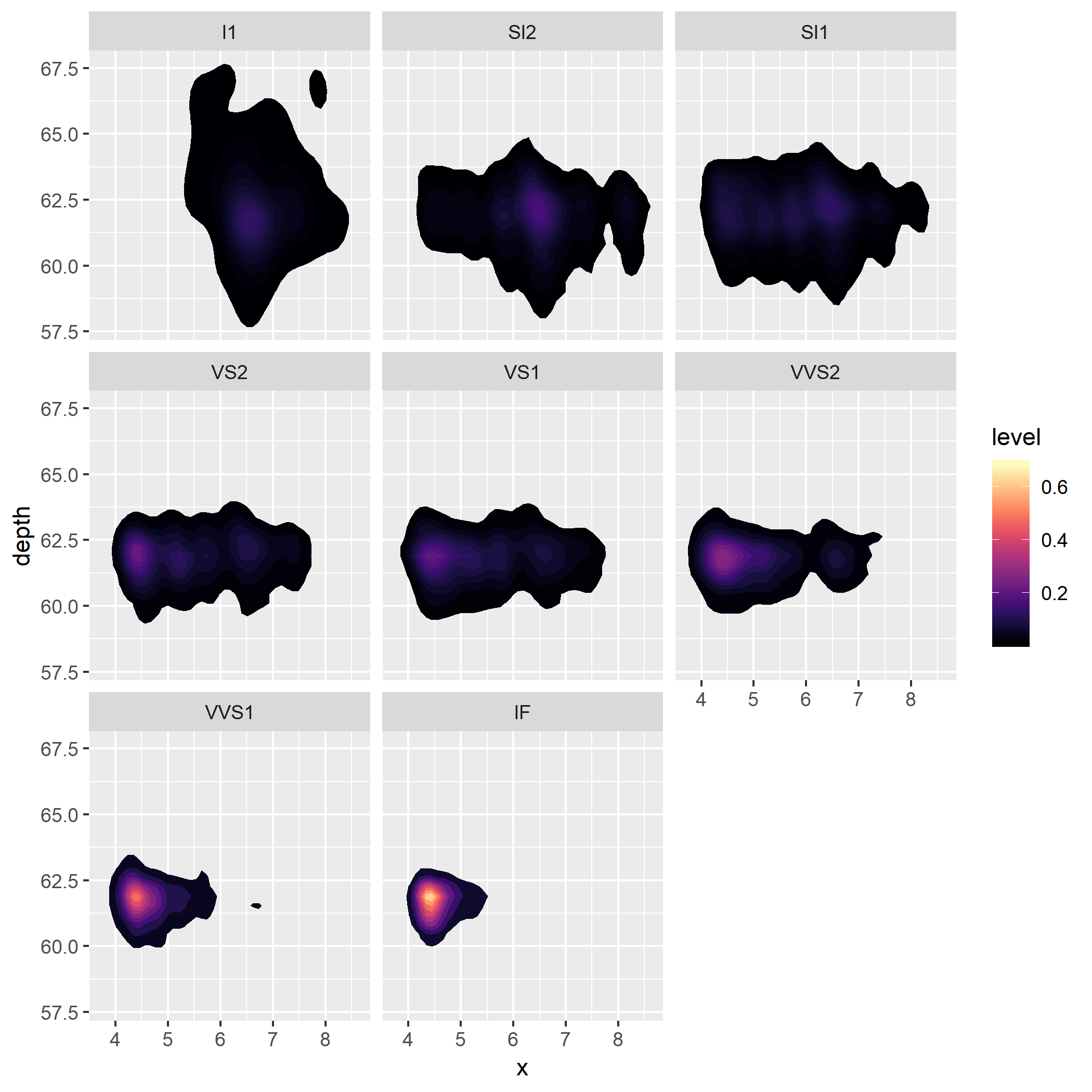As you can see, its hard to tell where the area of highest density is in all but VVS1 and IF. However, if I render the first panel alone, the area of highest density is very clear. ``````diamonds %>% filter(clarity == "I1") %>% ggplot(aes(x=x, y= depth)) + stat_density_2d(aes(fill = stat(level)), geom = "polygon", n = 100 , bins = 10) + facet_wrap(clarity~.) + scale_fill_viridis(option = "A") ``````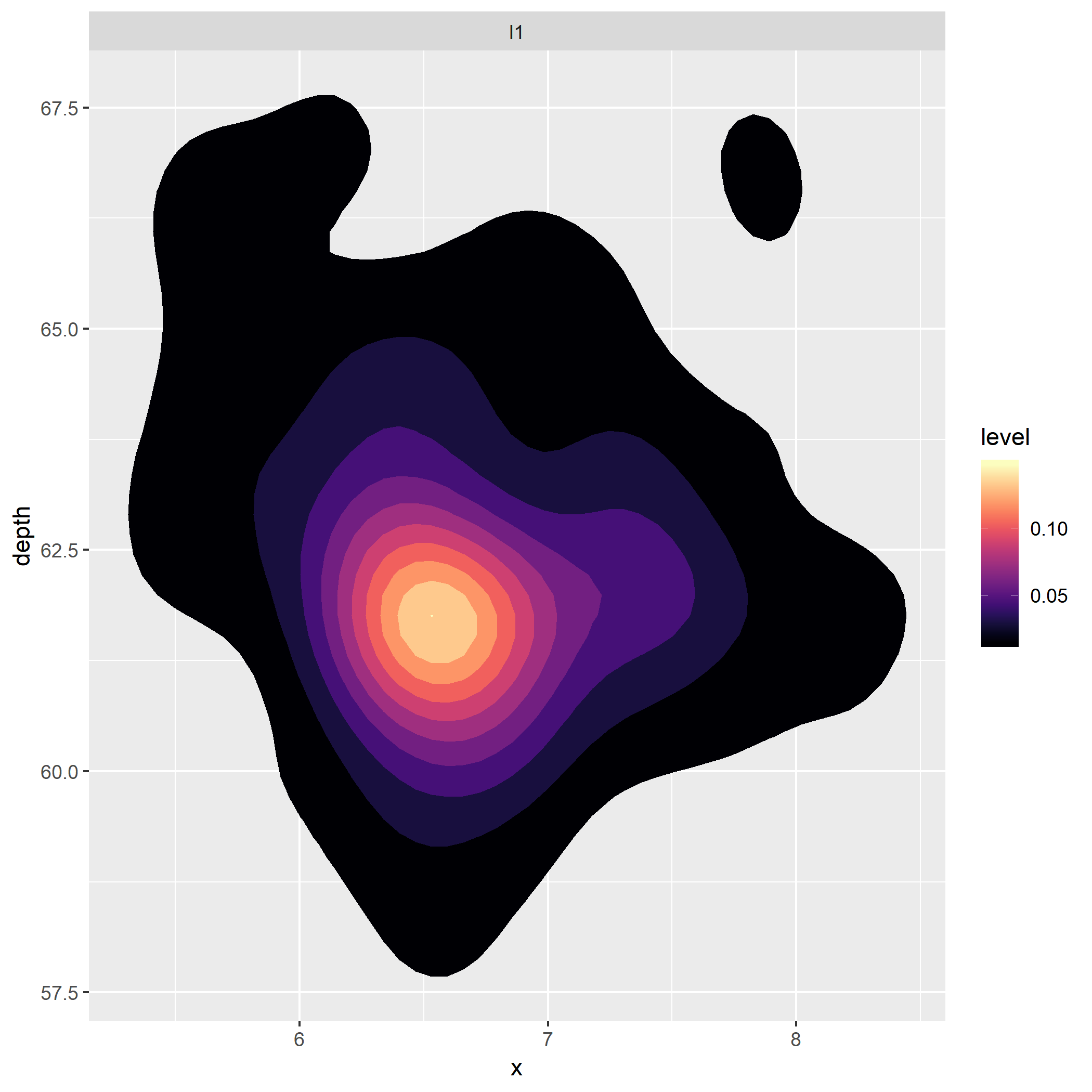If I set the fill to `stat(piece)` then I'm closer to achieving the right scaling; though there are still some panels which are not using the full color palette. (This has been my temporary solution.) ``````plot.denisty2d_piece <- diamonds %>% ggplot(aes(x=x, y= depth)) + stat_density_2d(aes(fill = stat(piece)), geom = "polygon", n = 100, bins = 10, contour = T) + facet_wrap(clarity~.) + scale_fill_viridis(option = "A") plot.denisty2d_piece ``````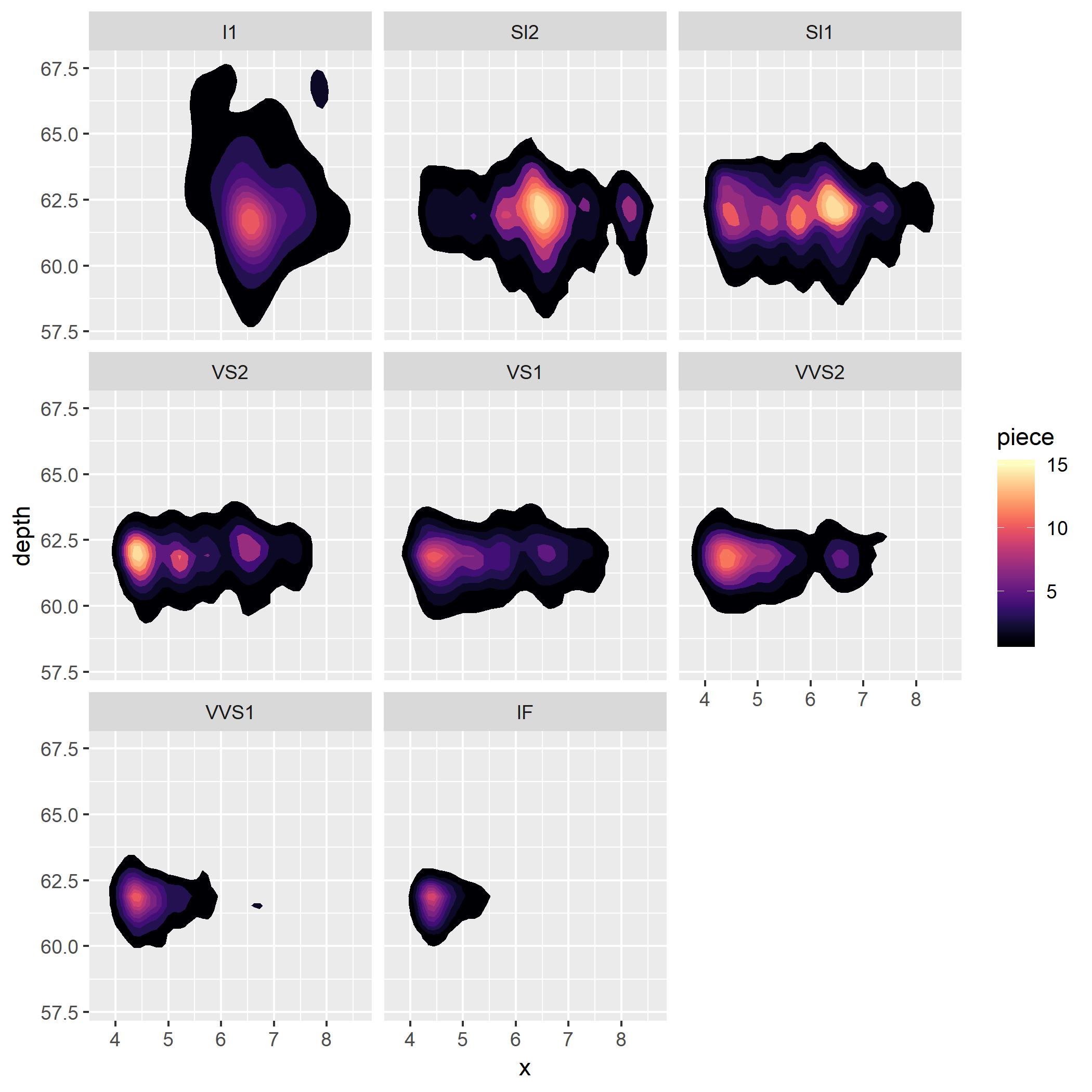`stat_bin2d` and `stat_hex` also lack the ability to set the fill to a 'scaled density' such as 'ndensity' or 'ncount' ``````plot.bin2d <- diamonds %>% ggplot(aes(x=x, y= depth)) + stat_bin2d(bins = 60, aes(fill = stat(count))) + facet_wrap(clarity~.) + scale_fill_viridis(option = "A") str(ggplot_build(plot.bin2d)\$data[]) #> 'data.frame': 2716 obs. of 20 variables: #> \$ fill : chr "#000004" "#000004" "#000004" "#000004" ... #> \$ xbin : int 27 49 38 48 54 34 42 43 48 53 ... #> \$ ybin : int 22 22 23 23 23 24 24 24 24 24 ... #> \$ value : num 1 1 1 1 1 2 1 1 1 1 ... #> \$ x : num 4.74 8.68 6.71 8.5 9.58 ... #> \$ y : num 55.5 55.5 56.1 56.1 56.1 ... #> \$ count : num 1 1 1 1 1 2 1 1 1 1 ... #> \$ density : num 0.00135 0.00135 0.00135 0.00135 0.00135 ... #> \$ PANEL : Factor w/ 8 levels "1","2","3","4",..: 1 1 1 1 1 1 1 1 1 1 ... #> \$ group : int -1 -1 -1 -1 -1 -1 -1 -1 -1 -1 ... #> \$ xmin : num 4.65 8.59 6.62 8.41 9.49 ... #> \$ xmax : num 4.83 8.77 6.8 8.59 9.67 ... #> \$ ymin : num 55.2 55.2 55.8 55.8 55.8 ... #> \$ ymax : num 55.8 55.8 56.4 56.4 56.4 ... #> \$ colour : logi NA NA NA NA NA NA ... #> \$ size : num 0.1 0.1 0.1 0.1 0.1 0.1 0.1 0.1 0.1 0.1 ... #> \$ linetype: num 1 1 1 1 1 1 1 1 1 1 ... #> \$ alpha : logi NA NA NA NA NA NA ... #> \$ width : logi NA NA NA NA NA NA ... #> \$ height : logi NA NA NA NA NA NA ... plot.hex <- diamonds %>% ggplot(aes(x=x, y= depth)) + stat_binhex(bins = 60, aes(fill = stat(count))) + facet_wrap(clarity~.) + scale_fill_viridis(option = "A") str(ggplot_build(plot.hex)\$data[]) #> 'data.frame': 3005 obs. of 10 variables: #> \$ fill : chr "#000004" "#000004" "#000004" "#000004" ... #> \$ x : num 4.74 8.5 8.68 6.62 9.49 ... #> \$ y : num 55.7 55.7 55.7 56.2 56.2 ... #> \$ density: num 0.00135 0.00135 0.00135 0.00135 0.00135 ... #> \$ count : int 1 1 1 1 1 2 1 1 1 1 ... #> \$ PANEL : Factor w/ 8 levels "1","2","3","4",..: 1 1 1 1 1 1 1 1 1 1 ... #> \$ group : int -1 -1 -1 -1 -1 -1 -1 -1 -1 -1 ... #> \$ colour : logi NA NA NA NA NA NA ... #> \$ size : num 0.5 0.5 0.5 0.5 0.5 0.5 0.5 0.5 0.5 0.5 ... #> \$ alpha : logi NA NA NA NA NA NA ... `````` Compare this to the 1d `stat_density` and `stat_histogram` which both provide the option to rescale each facet to a maxium of 1 using the `y = stat(scaled)` and `y = stat(ndensity)` option respectively. ``````plot.density <- diamonds %>% ggplot(aes(x=x)) + geom_density(aes(y = stat(scaled))) + facet_wrap(clarity~.) + scale_fill_viridis(option = "A") plot.density ``````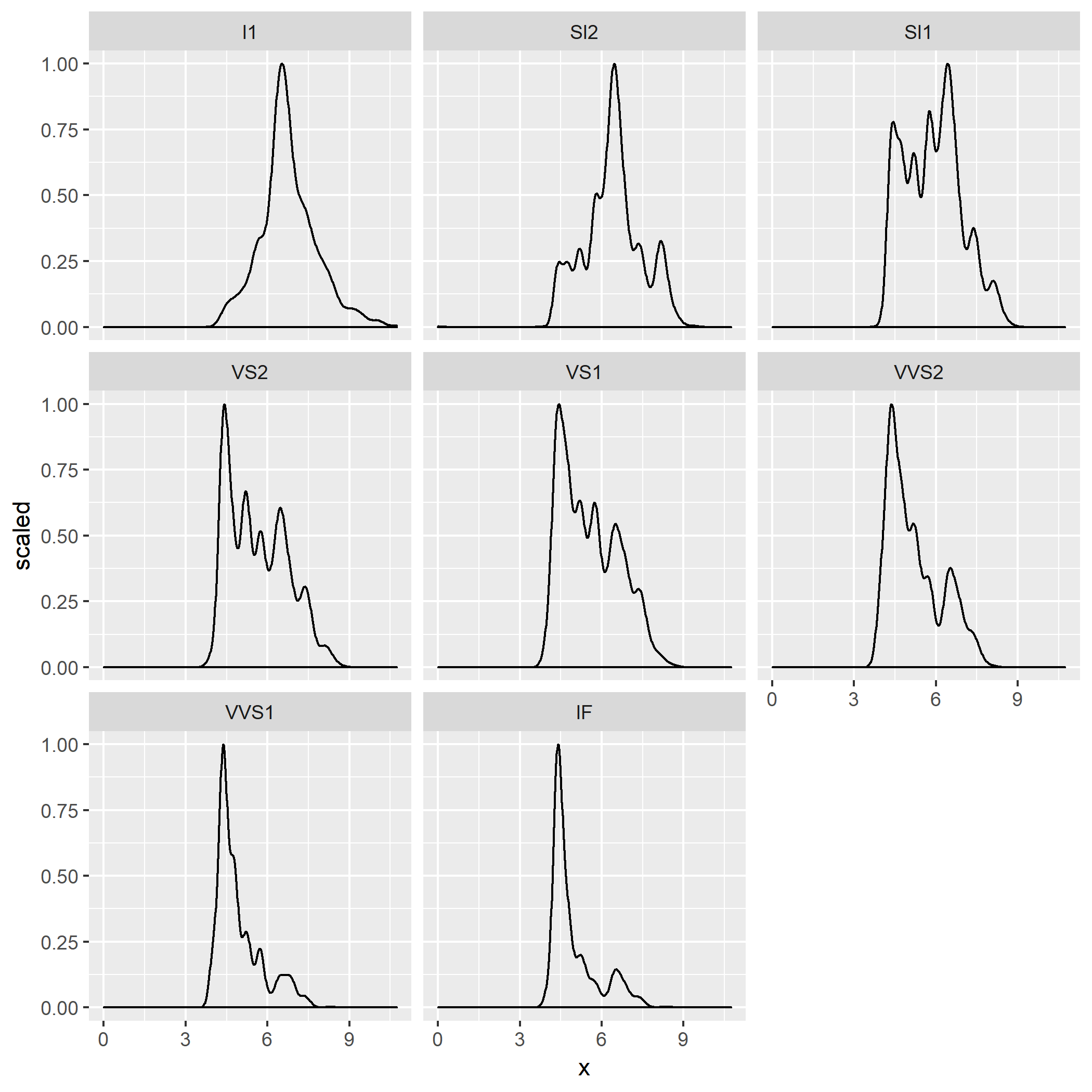``````plot.histogram <- diamonds %>% ggplot(aes(x=x)) + geom_histogram(bins = 60, aes(y = stat(ndensity))) + facet_wrap(clarity~.) + scale_fill_viridis(option = "A") plot.histogram ``````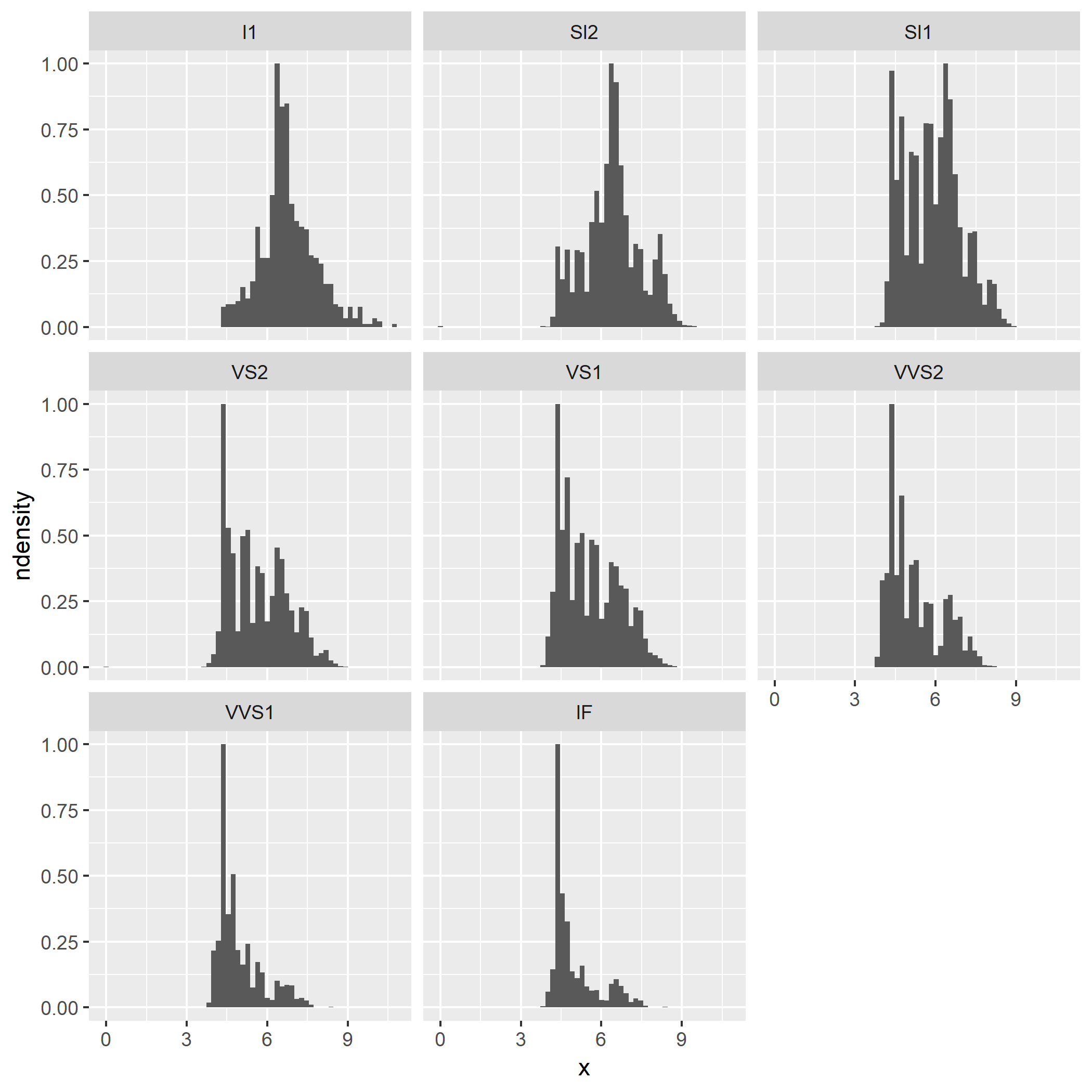Would you consider adding a "scaled" statistic to stat_density2d and an "ndensity/ncount" statistic to stat_bin2d and stat_binhex? The text was updated successfully, but these errors were encountered:
added a commit to bjreisman/ggplot2 that referenced this issue Jul 11, 2018
``` Fixes tidyverse#2679 ```
``` 814d0d1 ```
pushed a commit to bjreisman/ggplot2 that referenced this issue Jul 11, 2018
``` Fixes tidyverse#2679 ```
``` 7c64c91 ```

### lock bot commented Jan 7, 2019

 This old issue has been automatically locked. If you believe you have found a related problem, please file a new issue (with reprex) and link to this issue. https://reprex.tidyverse.org/